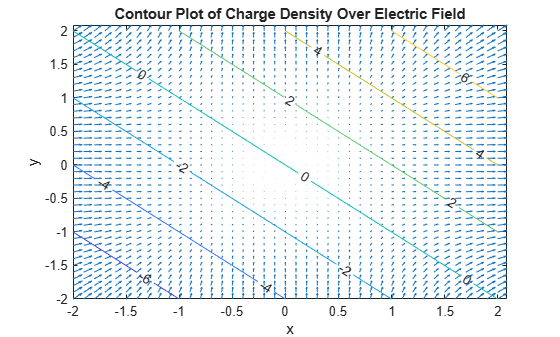# divergence

Divergence of symbolic vector field

## Syntax

``d = divergence(V,X)``
``d = divergence(V)``

## Description

example

````d = divergence(V,X)` returns the divergence of symbolic vector field `V` with respect to vector `X` in Cartesian coordinates. Vectors `V` and `X` must have the same length.```
````d = divergence(V)` returns the divergence of the vector field `V` with respect to a default vector constructed from the symbolic variables in `V`.```

## Examples

collapse all

Find the divergence of the vector field $\mathit{V}\left(\mathit{x},\mathit{y},\mathit{z}\right)=\left(\mathit{x},2{\mathit{y}}^{2},3{\mathit{z}}^{3}\right)$ with respect to vector $\mathit{X}=\left(\mathit{x},\mathit{y},\mathit{z}\right)$.

```syms x y z V = [x 2*y^2 3*z^3]; X = [x y z]; div = divergence(V,X)```
`div = $9 {z}^{2}+4 y+1$`

Show that the divergence of the curl of the vector field is 0.

`divCurl = divergence(curl(V,X),X)`
`divCurl = $0$`

Find the divergence of the gradient of the scalar field $\mathit{f}\left(\mathit{x},\mathit{y},\mathit{z}\right)={\mathit{x}}^{2}+{\mathit{y}}^{2}+{\mathit{z}}^{2}$. The result is the Laplacian of the scalar field.

```syms x y z f = x^2 + y^2 + z^2; divGrad = divergence(gradient(f,X),X)```
`divGrad = $6$`

Gauss’s Law in differential form states that the divergence of an electric field is proportional to the electric charge density.

`$\underset{}{\overset{\to }{\nabla }}\cdot \underset{}{\overset{\to }{E}}\left(\underset{}{\overset{\to }{r}}\right)=\frac{\rho \left(\underset{}{\overset{\to }{r}}\right)}{{ϵ}_{0}}$`

Find the electric charge density for the electric field $\underset{}{\overset{\to }{E}}={x}^{2}\underset{}{\overset{ˆ}{i}}+{y}^{2}\underset{}{\overset{ˆ}{j}}$.

```syms x y ep0 E = [x^2 y^2]; rho = divergence(E,[x y])*ep0```
`rho = ${\mathrm{ep}}_{0} \left(2 x+2 y\right)$`

Visualize the electric field and electric charge density for $-2<\mathit{x}<2$ and $-2<\mathit{y}<2$ with `ep0 = 1`. Create a grid of values of `x` and `y` using `meshgrid`. Find the values of the electric field and charge density by substituting grid values using `subs`. Simultaneously substitute the grid values `xPlot` and `yPlot` into the charge density `rho` by using cell arrays as inputs to `subs`.

```rho = subs(rho,ep0,1); v = -2:0.1:2; [xPlot,yPlot] = meshgrid(v); Ex = subs(E(1),x,xPlot); Ey = subs(E(2),y,yPlot); rhoPlot = double(subs(rho,{x,y},{xPlot,yPlot}));```

Plot the electric field using `quiver`. Overlay the charge density using `contour`. The contour lines indicate the values of the charge density.

```quiver(xPlot,yPlot,Ex,Ey) hold on contour(xPlot,yPlot,rhoPlot,"ShowText","on") title("Contour Plot of Charge Density Over Electric Field") xlabel("x") ylabel("y")```Since R2023a

Derive the electromagnetic wave equation in free space without charge and without current sources from Maxwell's equations.

First, create symbolic scalar variables to represent the vacuum permeability and permittivity. Create a symbolic matrix variable to represent the Cartesian coordinates. Create two symbolic matrix functions to represent the electric and magnetic fields as functions of space and time.

```syms mu_0 epsilon_0 syms X [3 1] matrix syms E(X,t) B(X,t) [3 1] matrix keepargs```

Next, create four equations to represent Maxwell's equations.

`Maxwell1 = divergence(E,X) == 0`
`Maxwell1(X, t) = ${\nabla }_{X}·E\left(X,t\right)={\mathrm{0}}_{1,1}$`
`Maxwell2 = curl(E,X) == -diff(B,t)`
```Maxwell2(X, t) =  ```
`Maxwell3 = divergence(B,X) == 0`
`Maxwell3(X, t) = ${\nabla }_{X}·B\left(X,t\right)={\mathrm{0}}_{1,1}$`
`Maxwell4 = curl(B,X) == mu_0*epsilon_0*diff(E,t)`
```Maxwell4(X, t) =  ```

Then, find the wave equation for the electric field. Compute the curl of the second Maxwell equation.

`wave_E = curl(Maxwell2,X)`
```wave_E(X, t) =  ```

Substitute the first Maxwell equation in the electric field wave equation. Use `lhs` and `rhs` to obtain the left and right sides of the first Maxwell equation.

`wave_E = subs(wave_E,lhs(Maxwell1),rhs(Maxwell1))`
```wave_E(X, t) =  ```

Compute the time derivative of the fourth Maxwell equation.

`dMaxwell4 = diff(Maxwell4,t)`
```dMaxwell4(X, t) =  ```

Substitute the term that involves the magnetic field ${\nabla }_{\mathbit{X}}×\frac{\partial }{\partial \mathit{t}}\mathbit{B}\left(\mathbit{X},\mathit{t}\right)$ in `wave_E` with the right side of `dMaxwell4`. Use `lhs` and `rhs` to obtain these terms from `dMaxwell4`.

`wave_E = subs(wave_E,lhs(dMaxwell4),rhs(dMaxwell4))`
```wave_E(X, t) =  ```

Using similar steps, you can also find the wave equation for the magnetic field.

`wave_B = curl(Maxwell4,X)`
```wave_B(X, t) =  ```
`wave_B = subs(wave_B,lhs(Maxwell3),rhs(Maxwell3))`
```wave_B(X, t) =  ```
`dMaxwell2 = diff(Maxwell2,t)`
```dMaxwell2(X, t) =  ```
`wave_B = subs(wave_B,lhs(dMaxwell2),rhs(dMaxwell2))`
```wave_B(X, t) =  ```

## Input Arguments

collapse all

Symbolic vector field, specified as a vector of symbolic scalar variables, symbolic function, symbolic matrix variable, or symbolic matrix function. `V` must have the same length as `X`.

• If `V` is a function of symbolic scalar variables, where `V` is of type `sym` or `symfun`, then the vector `X` must be of type `sym` or `symfun`.

• If `V` is a function of symbolic matrix variables, where `V` is of type `symmatrix` or `symfunmatrix`, then the vector `X` must be of type `symmatrix` or `symfunmatrix`.

Data Types: `sym` | `symfun` | `symmatrix` | `symfunmatrix`

Vector with respect to which you find the divergence, specified as a vector of symbolic scalar variables, symbolic function, symbolic matrix variable, or symbolic matrix function. `X` must have the same length as `V`.

• If you do not specify `X` and `V` is a function of symbolic scalar variables, then, by default, `divergence` constructs vector `X` from the symbolic scalar variables in `V` with the order of variables as defined by `symvar(V)`.

• If `X` is a symbolic matrix variable of type `symmatrix`, then `X` must have a size of `1`-by-`N` or `N`-by-`1`.

• If `V` and `X` are scalars, then `divergence(V,X) = diff(V,X)`.

Data Types: `sym` | `symfun` | `symmatrix` | `symfunmatrix`

## Limitations

• The `divergence` function does not support tensor derivatives. If the input `V` is a tensor field or a matrix rather than a vector, then the `divergence` function returns an error.

• Symbolic Math Toolbox™ currently does not support the `dot` or `cross` functions for symbolic matrix variables and functions of type `symmatrix` and `symfunmatrix`. If vector calculus identities involve dot or cross products, then the toolbox displays those identities in terms of other supported functions instead. To see a list of all the functions that support symbolic matrix variables and functions, use the commands ```methods symmatrix``` and `methods symfunmatrix`.

collapse all

### Divergence of Symbolic Vector Field

The divergence of the symbolic vector field V = (V1,...,Vn) with respect to the vector X = (X1,...,Xn) in Cartesian coordinates is the sum of partial derivatives of V with respect to X1,...,Xn.

`$div\left(\stackrel{\to }{V}\right)={\nabla }_{X}\cdot \stackrel{\to }{V}=\sum _{i=1}^{n}\frac{\partial {V}_{i}}{\partial {X}_{i}}$`

## Version History

Introduced in R2012a

expand all